March 4, 2021

# Introduction to differential topology and geometry

This is a list of books ordered so that you will feel comfortable later studying classical field theory, quantum field theory, string theory and general relativity (from a mathematical point of view)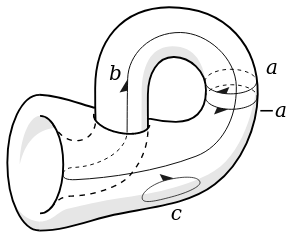Homology cycles on the surface of a Klein bottle. Credit: Steelpillow from wikipedia.

I have divided the “course” in three main blocks. Read the comments.

(1) FIRST BLOCK

★Algebra

“Algebra”, by Michael Artin. Englewood Cliffs, N.J.: Prentice Hall. 1991. 2nd edition. Boston: Pearson Education. 2011

Study the first chapter is on matrices and determinants (algebraic background to do exterior calculus). Read it in detail and then anything in the book related to groups and vector spaces. Fundamental for calculus on manifolds. Chapter 1 – 6 in particular are relevant, and 8. Read them in detail.

★Analysis

“Analysis I.” Third edition, by Terence Tao. Texts and Readings in Mathematics, 37. Hindustan Book Agency, New Delhi, 2014. xviii+347 pp. ISBN 978-93-80250-64-9

Good for self-studying. The methods he uses are general. Everything is done in the context of the 1-dimensional line, but in such a way that the techniques and thought process being used can be extended to general metric spaces. Consider excluding the Riemann integral chapter, last one, because it is out of the scope of this programme.

★Topology

“Topologie”, by Klaus Jänich. Springer-Verlag, 8. Auflage 2005, ISBN 978-3-540-21393-2, doi:10.1007/b138142 (Allgemeine Topologie, englische Übersetzung bei Undergraduate Texts in Mathematics, Springer-Verlag, 1984)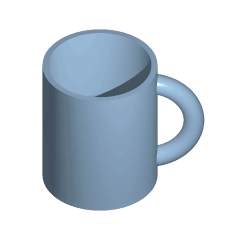Read in detail chapters I-IV. This book is like Lee’s book but more compact, which has pros and cons. It might be envisaged as an alternative or addition to Lee (see last one in the list). If you decide to pick up Lee’s, then you should read all of it including compactness, stopping there before moving to the 3rd block. If you have finished compactness, you can continue studying it in a parallel way as you move forward to the 3rd block.

In this sense, regard Janich’s as an introduction to Lee’s book. It stars from basic, topological definitions, nothing special, but it conveys a good, working knowledge of what a topological space is.

The first chapters based on general topology might be very difficult because it is more compact. Lee’s discusses in more detail. The author’s interest is on geometry and hence general topology is introduced quickly and in not as much detail.

In particular the first chapter might be difficult. Consider defaulting to Lee’s book again and again if you encounter difficulties. Chapter III is the most challenging one, since it covers constructing topological spaces via gluing. This is the most important one in topology.

(2) SECOND BLOCK

★Analysis

“Analysis II.”, by Terence Tao. Third edition. Texts and Readings in Mathematics, 38. Hindustan Book Agency, New Delhi, 2014. xvi+218 pp. ISBN 978-93-80250-65-6

This book with the topology of metric spaces. Then it continues with spaces of continuous functions, discussing approximation techniques (approximation by polynomials and trigonometric series). This is useful because it allows us to approximate “rough” functions with “well-behaved” functions.

The last three chapters are an introduction to modern multivariable calculus. He finishes with Lebeque’s integral as tool to introduce volume. It is a generalization of the Riemann integral but the proof is easier to understand.

★Algebra

“Algebra”, by Michael Artin. Englewood Cliffs, N.J.: Prentice Hall. 1991. 2nd edition. Boston: Pearson Education. 2011

Study all related to rings and representation theory. Chapter 9, 10 and 11. Eventually, but maybe not necessarily, consider studying the others, from 12 to the end.

★Differential Equations

“Ordinary Differential Equations” by Vladimir Arnold, 1978, The MIT Press ISBN 0-262-51018-9.

Study all of it. The author introduces manifolds and differential geometry and topology in terms of ordinary differential equations. This book is optional but it gives you a tremendous intuition on how to do mathematics in general. Consider combining this book with this other work by the same author:

“Mathematical Methods of Classical Mechanics”. Graduate Texts in Mathematics. 60 (2nd ed.), 1989. Springer. doi:10.1007/978-1-4757-2063-1. ISBN 978-1-4419-3087-3

(3) THIRD BLOCK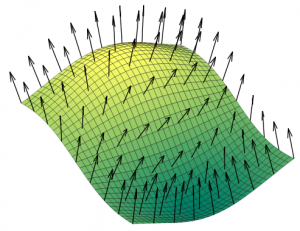The map associating a normal to each point on a surface can be thought of as a section. The surface is the space X, and at each point x there is a vector in the vector space attached at x.Credit: Nicoguaro.

★Differential Topology

“Introduction to Differential Topology” by Klaus Jänich and Theodor Bröcker, Cambridge University Press, 1982, ISBN 13: 9780521284707. Author: Brocker, Theodor ISBN 10: 0521284708.

Read it completely and in detail, because it is fundamental. You will need every single detail. Do not attempt to understand general relativity without this book. Do not move forward without it. Do not breathe without it.

★Analysis

“Analysis: Second Edition”, by Elliott H. Lieb and Michael Loss. Graduate Studies in Mathematics Volume: 14; 2001; 348 pp. Print ISBN: 978-0-8218-2783-3

Two mathematical relativists. This book can be described as an homage to approximation theories, in a rigorous fashion. It is connected to physics all throughout the book. The book presents techniques to be applied in (special) relativistic field theory and relativistic quantum mechanics. They also discuss classical problems (which does not mean “easy”) in the context of function analytical techniques.

★Lie Groups

“A Comprehensive Introduction to Differential Geometry”, by Michael Spivak. Publish or Perish; revised 3rd ed. 2005.

Study anything related to Lie groups from this book, which is basically one chapter. Without differential topology you will be lost for the rest of the programme.

★Geometry

“A Comprehensive Introduction to Differential Geometry”, by Michael Spivak, Volume 2. Publish or Perish; revised 3rd ed. 2005.

The author uses the original works by Gauss and Riemann and introduces them in a modern language, including the proofs. Spivak introduces in an intuitive way affine connections and the Riemann tensor. This is one of the best explanations of actual differential geometry in the literature. The book does not include exercises, though. One must resort to other books such as O’Niell, but studying Spivak’s proofs is probably enough for most of the contents.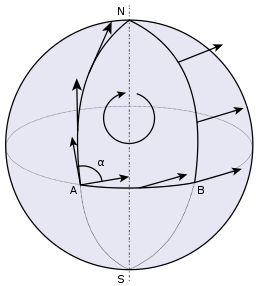Parallel transport of a vector around a closed loop (from A to N to B and back to A) on the sphere. The angle by which it twists, α, is proportional to the area inside the loop. Credit: Fred the Oyster

“Semi-Riemannian geometry. With applications to relativity”, by Barrett O’Niell. Pure and Applied Mathematics, 103. Academic Press, Inc. [Harcourt Brace Jovanovich, Publishers], New York, 1983. xiii+468 pp. ISBN 0-12-526740-1

The mathematician way of studying differential geometry to understand general relativity. The author uses a general approach, the concept of semi-Riemannian geometry, where we have inner product with no assumption on the signature. Lorentz and Riemann geometry are addressed in particular only when needed. i.e. specific cases are shown only in some cases.

★Complex Analysis

“Complex analysis. An introduction to the theory of analytic functions of one complex variable”, by Lars Ahlfors. Third edition. International Series in Pure and Applied Mathematics. McGraw-Hill Book Co., New York, 1978. xi+331 pp. ISBN 0-07-000657-1

This is crucial for three reasons. Firstly, many proofs in analysis relies on complex analysis. Secondly, many topological notions arise from complex analysis. The notions of homotopy and homology are related to the calculus of residues. For example, every orientable surface is constructed from Riemann surfaces (1-dimensional complex manifolds). They are common in quantum field theory and string theory in particular. They even come up when you are trying to calculate simple probability amplitudes, as you do this by analytic continuation, a subject related to Riemann surfaces.

★Topology

“Introduction to Topological Manifolds”, by John “Jack” Marshall Lee, Springer-Verlag, Graduate Texts in Mathematics 2000, 2nd edition 2011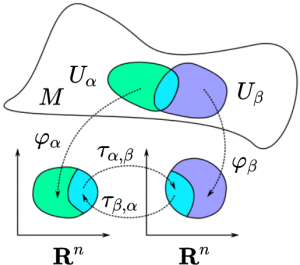Two charts on a manifold, and their respective transition map. Credit: Stomapoli

You will need it constantly, in particular when reading Janich’s, or when breaking down of compounds such of glucose to pyruvate by cellular respiration. I.e. when you undertake the pathway of the set of metabolic pathways that breaks down molecules into smaller units that are either oxidized to release energy or used in other anabolic reactions.